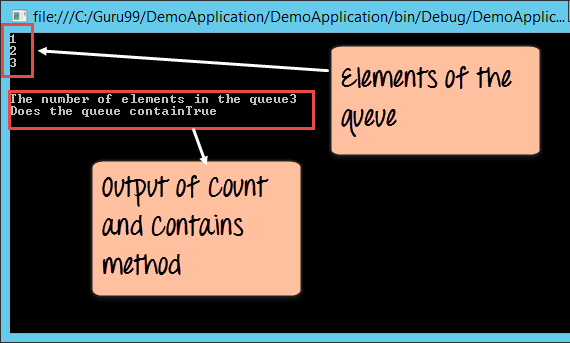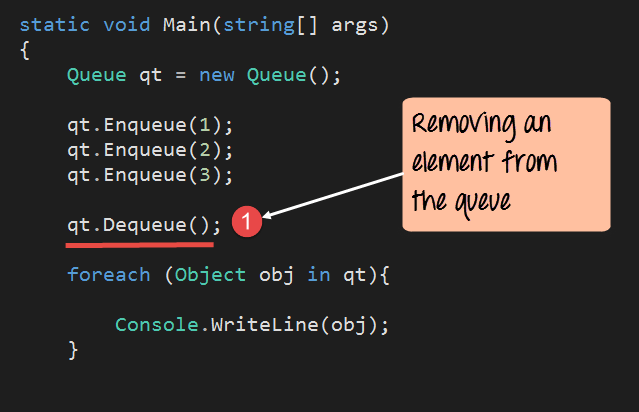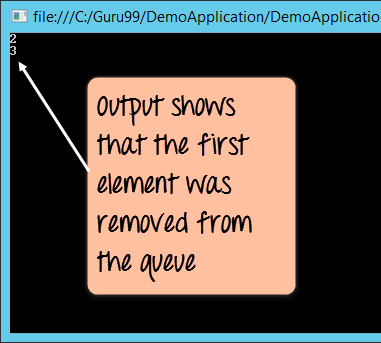# C# Queue

## What is Queue in C#?

The Queue is a special case collection which represents a first in first out concept. Imagine a queue of people waiting for the bus. Normally, the first person who enters the queue will be the first person to enter the bus. Similarly, the last person to enter the queue will be the last person to enter into the bus. Elements are added to the queue, one on the top of each other.

The process of adding an element to the queue is the enqueuer operation. To remove an element from a queue, you can use the dequeuer operation. The operation in queues is similar to stack we saw previously.

Let’s look at the operations available for the Queue collection in more detail.

### Declaration of the Queue

The declaration of a Queue is provided below. A Queue is created with the help of the Queue Data type. The “new” keyword is used to create an object of a Queue. The object is then assigned to the variable qt.

`Queue qt = new Queue()`

### Adding elements to the Queue

The enqueue method is used to add an element onto the queue. The general syntax of the statement is given below.

`Queue.enqueue(element)`

### Removing elements from the queue

The dequeue method is used to remove an element from the queue. The dequeue operation will return the first element of the queue. The general syntax of the statement is given below

`Queue.dequeue()`

### Count

This property is used to get the number of items in the queue. Below is the general syntax of this statement.

`Queue.Count`

### Contains

This method is used to see if an element is present in the Queue. Below is the general syntax of this statement. The statement will return true if the element exists, else it will return the value false.

`Queue.Contains(element)`

Now, let’s see this working at a code level. All of the below-mentioned code will be written to our Console application.

The code will be written to our Program.cs file. In the below program, we will write the code to see how we can use the above-mentioned methods.

### Example

In this example, we will see how a queue gets created. Next, we will see how to display the elements of the queue, and use the Count and Contain methods.```using System;
using System.Collections;
using System.Collections.Generic;
using System.Linq;
using System.Text;

namespace DemoApplication
{
class Program
{
static void Main(string[] args)
{
Queue qt = new Queue();
qt.Enqueue(1);
qt.Enqueue(2);
qt.Enqueue(3);

foreach (Object obj in qt)
{
Console.WriteLine(obj);
}
Console.WriteLine(); Console.WriteLine();
Console.WriteLine("The number of elements in the Queue " + qt.Count);
Console.WriteLine("Does the Queue contain " + qt.Contains(3));
}
}
} ```

### Code Explanation

1. The first step is used to declare the Queue. Here we are declaring qt as a variable to hold the elements of our Queue.
2. Next, we add 3 elements to our Queue. Each element is added via the “enqueue” method.
3. Now one thing that needs to be noted about Queues is that the elements cannot be accessed via the index position like the array list. We need to use a different approach to display the elements of the Queue. So here’s how we go about displaying the elements of a queue.
• We first declare a temporary variable called obj. This will be used to hold each element of the Queue.
• We then use the foreach statement to go through each element of the Queue.
• For each Queue element, the value is assigned to the obj variable.
• We then use the Console.Writeline command to display the value to the console.
1. We are using the “Count” property to get the number of items in the Queue. This property will return a number. We then display this value to the console.
2. We then use the “Contains” method to see if the value of 3 is present in our Queue. This will return either a true or false value. We then display this return value to the console.

If the above code is entered properly and the program is run the following output will be displayed.

### OutputFrom the output, we can clearly see that the elements of the Queue are displayed. Note that, unlike “stack” in “queue” the first element pushed on to the queue is displayed first. The count of queue elements is also shown in the output. Also, the value of True is displayed to say that the value of 3 is defined on the queue.

## C# Queue Dequeue

Now let’s look at the remove functionality. We will see the code required to remove the last element from the queue.```using System;
using System.Collections;
using System.Collections.Generic;
using System.Linq;
using System.Text;

namespace DemoApplication
{
class Program
{
static void Main(string[] args)
{
Queue qt = new Queue();
qt.Enqueue(1);
qt.Enqueue(2);
qt.Enqueue(3);

qt.Dequeue();

foreach (Object obj in qt)
{
Console.WriteLine(obj);
}
}
}
} ```

### Code Explanation

1. Here we just issue the “dequeue” method, which is used to remove an element from the queue. This method will remove the first element of the queue.

If the above code is entered properly and the program is run the following output will be displayed.

### Output:From the output, we can see that the first element which was added to the queue, which was the element 1, was removed from the queue.

Summary

• A Queue is based on the first in first out concept. The operation of adding an element to the queue is called the enqueue operation. The operation of removing an element to the queue is called the dequeue operation.